### Sample Problem

What is the equivalent capacitance of the following circuit?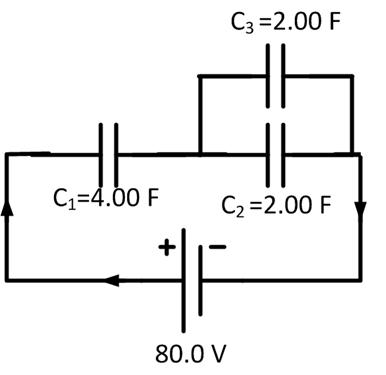#### Solution

First, we need to calculate the equivalent capacitance of the parallel section of the circuit.

C2 + 3 = 2.00F + 2.00F = 4.00F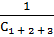=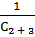+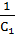=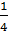+=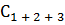= 2.00 F